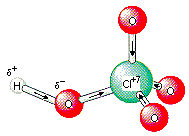Factors the Control the Relative Strengths of Acids and Bases

Factors that Control the Relative Strengths of Acids and Bases

The Polarity of the XH Bond

When all other factors are kept constant, acids become stronger as the XH bond becomes more polar. The second-row nonmetal hydrides, for example, become more acidic as the difference between the electronegativity of the X and H atoms increases. HF is the strongest of these four acids, and CH4 is one of the weakest Brnsted acids known.

 HF Ka = 7.2 x 10-4EN = 1.8 H2O Ka = 1.8 x 10-16EN = 1.2 NH3 Ka = 1 x 10-33EN = 0.8 CH4 Ka = 1 x 10-49EN = 0.4

When these compounds act as an acid, an H-X bond is broken to form H+ and X- ions. The more polar this bond, the easier it is to form these ions. Thus, the more polar the bond, the stronger the acid.

An 0.1 M HF solution is moderately acidic. Water is much less acidic, and the acidity of ammonia is so small that the chemistry of aqueous solutions of this compound is dominated by its ability to act as a base.

 HF pH = 2.1 H2O pH = 7 NH3 pH = 11.1The Size of the X Atom

At first glance, we might expect that HF, HCl, HBr, and HI would become weaker acids as we go down this column of the periodic table because the X-H bond becomes less polar. Experimentally, we find the opposite trend. These acids actually become stronger as we go down this column.

This occurs because the size of the X atom influences the acidity of the X-H bond. Acids become stronger as the X-H bond becomes weaker, and bonds generally become weaker as the atoms get larger as shown in the figure below.The Ka data for HF, HCl, HBr, and HI reflect the fact that the X-H bond-dissociation enthalpy (BDE) becomes smaller as the X atom becomes larger.

 HF Ka = 7.2 x 10-4 BDE = 569 kJ/mol HCl Ka = 1 x 106 BDE = 431 kJ/mol HBr Ka = 1 x 109 BDE = 370 kJ/mol HI Ka = 3 x 109 BDE = 300 kJ/molThe charge on a molecule or ion can influence its ability to act as an acid or a base. This is clearly shown when the pH of 0.1 M solutions of H3PO4 and the H2PO4-, HPO42-, and PO43- ions are compared.

 H3PO4 pH = 1.5 H2PO4- pH = 4.4 HPO42- pH = 9.3 PO43- pH = 12.0

Compounds become less acidic and more basic as the negative charge increases.

Acidity: H3PO4 > H2PO4- > HPO42-

Basicity: H2PO4- < HPO42- < PO43-There is no difference in the polarity, size, or charge when we compare oxyacids of the same element, such as H2SO4 and H2SO3 or HNO3 and HNO2, yet there is a significant difference in the strengths of these acids. Consider the following Ka data, for example.

 H2SO4: Ka = 1 x 103 HNO3: Ka = 28 H2SO3: Ka = 1.7 x 10-2 HNO2: Ka = 5.1 x 10-4

The acidity of these oxyacids increases significantly as the oxidation state of the central atom becomes larger. H2SO4 is a much stronger acid than H2SO3, and HNO3 is a much stronger acid than HNO2. This trend is easiest to see in the four oxyacids of chlorine.

 Oxyacid Ka Oxidation Number of the Chlorine HOCl 2.9 x 10-8 +1 HOClO 1.1 x 10-2 +3 HOClO2 5.0 x 102 +5 HOClO3 1 x 103 +7

This factor of 1011 difference in the value of Ka for hypochlorous acid (HOCl) and perchloric acid (HOClO3) can be traced to the fact that there is only one value for the electronegativity of an element, but the tendency of an atom to draw electrons toward itself increases as the oxidation number of the atom increases.

As the oxidation number of the chlorine atom increases, the atom becomes more electronegative. This tends to draw electrons away from the oxygen atoms that surround the chlorine, thereby making the oxygen atoms more electronegative as well, as shown in the figure below. As a result, the O-H bond becomes more polar, and the compound becomes more acidic.Practice Problem 6:For each of the following pairs, let's predict which compound is the stronger acid. (a) H2O or NH3 (b) NH4+ or NH3 (c) NH3 or PH3 (d) H3PO4 or H3PO3 Click here to check your answer to Practice Problem 6

The relative strengths of Brnsted bases can be predicted from the relative strengths of their conjugate acids combined with the general rule that the stronger of a pair of acids always has the weaker conjugate base.

 Practice Problem 7: For each of the following pairs of compounds, predict which compound is the stronger base. (a) OH- or NH2- (a) OH- or NH2- (c) NH2- or PH2- (d) NO3- or NO2- Click here to check your answer to Practice Problem 7